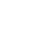Live chatIs given by sum of values in a set of data divided by number of values appearing in a set of data

The mean is equal to the sum of all the values in the data set divided by the number of values in the data set

or

= 52986 = 1261.57

42

Therefore the mean number of chocolate chips per bag is 1,262 (to the nearest whole number)

## Normal probability plot

No, it is not reasonable.

The mean is vulnerable and influenced by outliers, which are unusual values. If you compare its value to others in a set of data, you may end up with values that are too small or too large.

## From the charts above, there are no outliers

95% confidence interval for the mean (Note: Mean=1261.6; s=117.6)

((1261.6 - (1.96*117.6)), ((1261.6 + (1.96*117.6))

= (1031.1, 1492.1)

0

Preparing Orders

0

Active Writers

0%

Positive Feedback

0

Support Agents

Now Accepting Apple Pay!
Limited offerget 15% off your 1st order with code first15